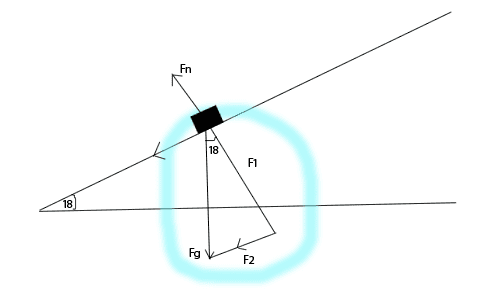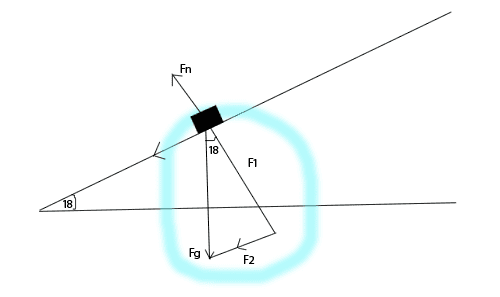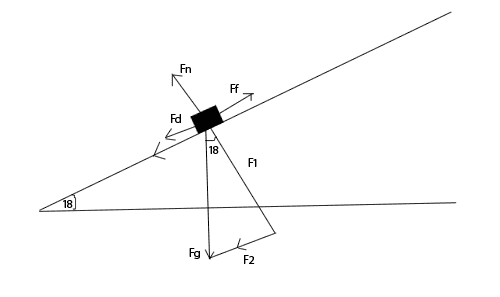# Measuring Coefficients of Friction (1 Viewer)

### Users Who Are Viewing This Thread (Users: 0, Guests: 1)

#### Dirkpitt

first off I'd like say thanks to everyone on here, I've lurked and solved many questions thanks to you guys. First post woo.

Basically we were tasked to design and carryout a lab to measure the coefficients of friction between a wooden board and some random objects (teachers gone for a few days so busy work basically). Easy stuff really, but I'm getting all confused with the components and way to solve for the coefficients/distinguish difference between solving for kinetic and static (if there is one).

1. The problem statement, all variables and given/known data

Have a bunch of angles but only need some help on getting started.

Object 1 - Static 18 degrees to start moving, kinetic 13.6 degrees to move at a constant velocity.

2. Relevant equations

This is where I'm lost basically.

3. The attempt at a solutionThis is the diagram I have. Excuse my 5 minute pentool job XD. With similar triangles the angle is 18 degrees, and I know you have to use cos and sin to make up for the "F1, F2" variables. Cos for F1, and Sin for F2, all I'm missing is how to use them. Thus I haven't gotten too far yet :(

From my current understanding, Fn = Fg - Ff. So Normal force has to equal the force of gravity minus the force of friction for the object to move, I'm just not sure on how to add in the F1 and F2. I'm thinking F=mg would work, and setting them equal to each other?

Something like;

F1=M(Gcos)
F2=M(Gsin)

Then M(Gcos)=M(Gsin), you could take out the "M", since M=M.

Gcos=Gsin
G=Sin/Cos
G=Tan

Honestly I don't really know where I was going with that, other then taking out the M :/

#### PhanthomJay

Homework Helper
Gold Member
first off I'd like say thanks to everyone on here, I've lurked and solved many questions thanks to you guys. First post woo.

Basically we were tasked to design and carryout a lab to measure the coefficients of friction between a wooden board and some random objects (teachers gone for a few days so busy work basically). Easy stuff really, but I'm getting all confused with the components and way to solve for the coefficients/distinguish difference between solving for kinetic and static (if there is one).

1. The problem statement, all variables and given/known data

Have a bunch of angles but only need some help on getting started.

Object 1 - Static 18 degrees to start moving, kinetic 13.6 degrees to move at a constant velocity.

2. Relevant equations

This is where I'm lost basically.

3. The attempt at a solutionThis is the diagram I have. Excuse my 5 minute pentool job XD. With similar triangles the angle is 18 degrees, and I know you have to use cos and sin to make up for the "F1, F2" variables. Cos for F1, and Sin for F2, all I'm missing is how to use them. Thus I haven't gotten too far yet :(
yes, F1 = mg cos theta, and F2 = mg sin theta. Those are the componets of mg. So now cross out mg in your free body diagram, because you already broke it up into its vector components.
From my current understanding, Fn = Fg - Ff. So Normal force has to equal the force of gravity minus the force of friction for the object to move,
Don't solve it by adding vectors, rather, solve it by adding vector components. Choose the incline as the x axis, and the normal force then acts along the y axis. Please label the friction force in your diagram, and show its direction. Now use Newton's Law (which one?) in the x and y direction, separately.

#### DirkpittAdding in Ff, which would be going up the incline, opposite to the direction of Fd. So Fnet=Fd-Ff, which is where I was earlier. Then from that, I've broken Fd into it's components and now I'm stumped.

I'm still getting lost in the equation part. I just don't know what the coefficient of friction is made up of. I feel like this is just a lack of my understanding of solving vector components. I've done that before, and vector addition, but in those cases there was always clear sets of x and y to be found. In this there is only F1 and F2, a single x and y.

Also to solve with newton's laws, you would use the second one? F=ma or F=mg?

Thanks for the help.

#### PhanthomJay

Homework Helper
Gold MemberAdding in Ff, which would be going up the incline,
yes, parallel to the incline
opposite to the direction of Fd. So Fnet=Fd-Ff, which is where I was earlier. Then from that, I've broken Fd into it's components and now I'm stumped.
What is Fd? The force acting opposite to Ff is F2, where F2 is mgsin theta, acting on the object . So in the x direction (take the x direction as parallel to the incline), it's F_net = F2 - Ff, but what is F_net if the object is not moving, or moving at constant velocity?
I'm still getting lost in the equation part. I just don't know what the coefficient of friction is made up of. I feel like this is just a lack of my understanding of solving vector components. I've done that before, and vector addition, but in those cases there was always clear sets of x and y to be found. In this there is only F1 and F2, a single x and y.
You know the force of friction is (mu_s)(N) when the object is just about to move, and (mu_k)(N) when it is moving relative to the incline, right? What is the value of N?
Also to solve with newton's laws, you would use the second one? F=ma or F=mg?

Thanks for the help.
If the object is not moving, or moving with constant velocity, F_net = ????

### The Physics Forums Way

We Value Quality
• Topics based on mainstream science
• Proper English grammar and spelling
We Value Civility
• Positive and compassionate attitudes
• Patience while debating
We Value Productivity
• Disciplined to remain on-topic
• Recognition of own weaknesses
• Solo and co-op problem solving